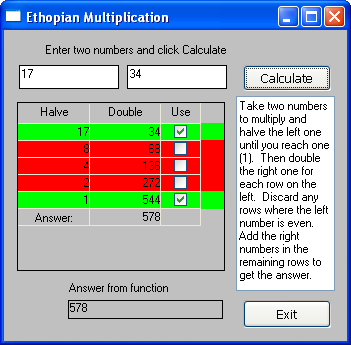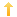## PowerBuilder – Ethiopian multiplication

Posted on Friday, August 20th, 2010 at 8:01 pm in

I stumbled across this task on the Rosetta Code website. (Link here) Of course I decided to do the task and, as a result, added Powerbuilder to the Rosetta Code website list of languages. There are plenty of other tasks to do so get crackin’.

The process of this method of multiplication is to write down the numbers to be multiplied in two columns. Take the left number and halve it (dropping fractions) and put that number as a new entry in the column until you reach one (1). Then take the second (rightmost) number and double it for every row on the left. Cross out any rows where the left column number is even. Add the remaining numbers from the right column to obtain the answer. The link above to Rosetta Code shows this process in a concise manner.

The task is to create four functions.

Function one determines if a number is even.
Function two halves a number.
Function three doubles a number.
Function four puts them all together to perform the process.

```public function boolean wf_iseven (long al_arg);
return mod(al_arg, 2 ) = 0
end function

public function long wf_halve (long al_arg);
RETURN int(al_arg / 2)
end function

public function long wf_double (long al_arg);
RETURN al_arg + al_arg
end function

public function long wf_ethiopianmultiplication (long al_multiplicand, long al_multiplier);
// calculate result
long ll_product

DO WHILE al_multiplicand >= 1
IF wf_iseven(al_multiplicand) THEN
// do nothing
ELSE
ll_product += al_multiplier
END IF
al_multiplicand = wf_halve(al_multiplicand)
al_multiplier = wf_double(al_multiplier)
LOOP

return ll_product
end function```

I also wrote a simple app to show the process via a datawindow and to perform the function call. The datawindow example makes use of expressions to determine which rows to use to obtain the final answer.The example .pbl is written in pb11.5 but it includes exports of the objects so you can bring it back to whatever version you are using.
eth_multiplication.zip file

Tags: